# SBI PO Quantitative Aptitude Questions 2019 (Day-37) High Level New Pattern

Dear Aspirants, the most awaited notification of SBI PO – 2019 has been released. We all know that new pattern questions are introducing every year in the SBI PO exam. Further, the questions are getting tougher and beyond the level of the candidate’s expectations.

Our IBPS Guide is providing High-Level New Pattern Quantitative Aptitude Questions for SBI PO 2019 so the aspirants can practice it on a daily basis. These questions are framed by our skilled experts after understanding your needs thoroughly. Aspirants can practice these high-level questions daily to familiarize with the exact exam pattern. We wish that your rigorous preparation leads you to a successful target of becoming SBI PO.

[WpProQuiz 5990]

### Click Here for SBI PO Pre 2019 High-Quality Mocks Exactly on SBI Standard

Directions (1 – 5): Study the following information carefully and answer the questions given below:

There are 10 mixtures A, B, C, D, E, F, G, H, I and J of Petrol and Diesel. Read the data given in the graphs.

Bar graph given below shows the amount of Petrol in first 5 mixtures A, B, C, D and E as a per cent of amount of Diesel in these mixtures.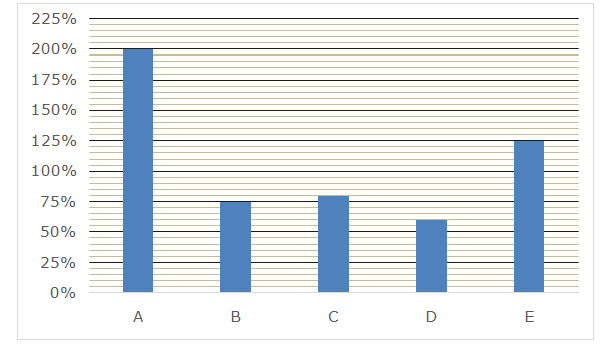Line graph given below shows the amount of Diesel in last 5 mixtures F, G, H, I and J as a per cent of amount of Petrol in these mixtures.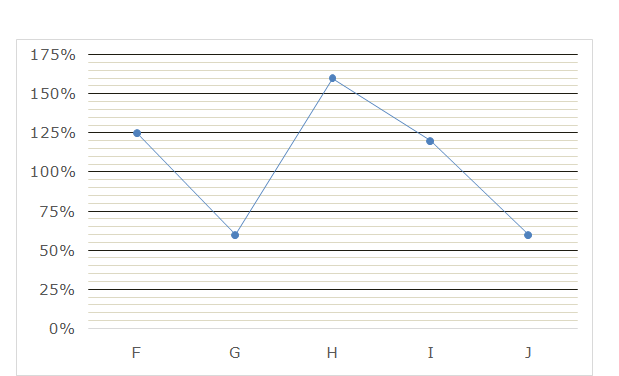1) If mixtures A and C are mixed in the ratio 1: 9 to form mixture P and mixtures B and D are mixed in the ratio 7: 48 to form mixture Q. Finally, mixtures P and Q are mixed in the ratio 3: 11 to form a final mixture, then total amount of Diesel in the final mixture is what per cent of total amount of Petrol in it?

a) 166(2/3)%

b) 150%

c) 87(1/3)%

d) 75%

e) None of these

2) If the total quantity of mixtures F, G and H is 45 litres, 80 litres and 130 litres respectively. If all the three mixtures F, G and H are poured into a large container and ‘x’ litres of Petrol is added to this large container, then what is the value of ‘x’ so that amount of Diesel in large container becomes 45% of total volume?

a) 60 litres

b) 30 litres

c) 90 litres

d) 45 litres

e) None of these

3)If the difference between the quantity of Petrol and Diesel in the mixture E is 40 litres and ‘x’ litres of mixture J is mixed with the total quantity of this mixture E to form a final mixture. Now, the ratio of Petrol to Diesel in the final mixture becomes 15: 11. Then what is the difference between the quantity of Petrol and Diesel in the mixture J?

a) 48 litres

b) 40litres

c) 30 litres

d) 45 litres

e) None of these

4) If the ratio of total amount of mixtures E, F and G is 6: 9: 8 respectively and total amount of Petrol in mixture G is 70 litres more than the amount of Diesel in mixture E. If all the three mixtures are poured into a large cubical tank of length 230 cm, width 150 cm and height 25 cm, then find the height of empty part of the tank?

a) 6 cm

b) 8 cm

c) 10 cm

d) 5 cm

e) 15 cm

5) If the ratio of quantity of Petrol in mixture D to that in mixture H is 9: 5 and difference between quantity of Petrol and Diesel in mixture D is 30 litres. What is the difference between the total quantity of Petrol and total quantity of Diesel in the final mixture if mixtures D and H are mixed together to form final mixture?

a) 60 litres

b) 30 litres

c) 50 litres

d) 75 litres

e) None of these

Directions (6 – 10): The questions below are based on the given Series-I. The series-I satisfy a certain pattern, follow the same pattern in Series-II and answer the questions given below.

6) I. 3256, 3260, 3251, 3276, 3227, 3348

II. 1089 …… 1301. If 1301 is the nth term, then find the value of n?

a) 5

b) 6

c) 7

d) 9

e) 8

7) I. 184, 356, 969, 2207, 4286, 7454

II. 42, 211 …. 4132. If 4132 is the nth term, what value should come in the place of (n+1)th term?

a) 2207

b) 8117

c) 9207

d) 7297

e) 7587

8) I. 179, 195, 212, 248, 359, 807

II. 192, 211…… 922. If 922 is the nth term, then find the value of n?

a) 11

b) 10

c) 9

d) 7

e) 6

9) I. 64, 33, 34, 52, 105, 263.5

II. 72 ….. 881.5. If 881.5 is the nth term, then what value should come in the place of (n-2)th term?

a) 117

b) 38

c) 58

d) 293.5

e) 457.5

10) I. 722, 850, 994, 1202, 1538, 2386

II. 84, 256, 446 ….. 4230. If 4230 is the nth term, find the value of (n+1)?

a) 11

b) 10

c) 9

d) 8

e) 7

Directions (1 – 5)

Bar graph shows the amount of Petrol as a per cent of amount of diesel. Let consider amount of diesel in first 5 mixtures A, B, C, D and E is 100 each.

Line graph shows the amount of Diesel as a per cent of amount of petrol. Let consider amount of petrol in last 5 mixtures F, G, H, I and J is 100 each.

 Mixtures Ratio (Petrol: Diesel) Mixtures Ratio (Petrol: Diesel) A 200: 100 2: 1 F 100: 125 4: 5 B 75: 100 3: 4 G 100: 60 5: 3 C 80: 100 4: 5 H 100: 160 5: 8 D 60: 100 3: 5 I 100: 120 5: 6 E 125: 100 5: 4 J 100: 60 5: 3

Let part of Petrol in mixture P and Q is ‘P’ and ‘Q’ respectively.

Mixtures A and C are mixed in the ratio 1: 9 to form mixture P:

By the rule of alligation: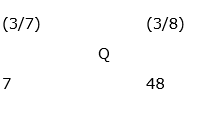[(4/9) – P]: [P – (2/3)] = 1: 9

4 – 9P = P – (2/3)

10P = (14/3)

P = 14/30 = 7/15

Mixtures B and D are mixed in the ratio 7: 48 to form mixture Q:

By the rule of alligation: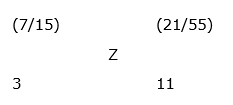[(3/8) – Q]: [Q – (3/7)] = 7: 48

18 – 48Q = 7Q – 3

55Q = 21

Q = 21/55

Let part of Petrol in final mixture = Z

Mixtures P and Q are mixed in the ratio 3: 11 to form mixture Q:

By the rule of alligation:

[(21/55) – Z]: [Z – (7/15)] = 3: 11

4.2 – 11Z = 3Z – 1.4

14Z = 5.6

Z = 5.6/14 = 2/5

Ratio of Petrol to Diesel in the final mixture = 2: (5 – 2) = 2: 3

Required per cent = (3/2) * 100 = 150%

In 45 litres of mixture F,

Petrol = 45 * (4/9) = 20 litres

Diesel = 45 – 20 = 25 litres

In 80 litres of mixture G,

Petrol = 80 * (5/8) = 50 litres

Diesel = 80 – 50 = 30 litres

In 130 litres of mixture H,

Petrol = 130 * (5/13) = 50 litres

Diesel = 130 – 50 = 80 litres

Total amount of Petrol in large container = 20 + 50 + 50 + x = (120 + x)

Total amount of Diesel in large container = 25 + 30 + 80 = 135

Total volume of mixture in the large container = (120 + x) + 135 = (255 + x)

According to the question:

[135/(255 + x)] = 45/100 = 9/20

2700 = 2295 + 9x

9x = 405

x = 45 litres

Let quantity of Petrol and Diesel in mixture E is 5a and 4a respectively.

According to the question:

5a – 4a = 40

a = 40

Quantity of Petrol in mixture E = 5a = 200 litres

Quantity of Diesel in mixture E = 4a =160 litres

Total quantity of mixture E = 200 + 160 = 360 litres

By the rule of alligation: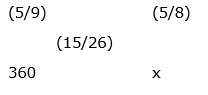[(5/8) – (15/26)]: [(15/26) – (5/9)] = 360: x

9: 4 = 360: x

x = (360 * 4)/9

x = 160

Quantity of Petrol in mixture J = 160 * (5/8) = 100 litres

Quantity of Diesel in mixture J = 160 – 100 = 60 litres

Required difference = 100 – 60 = 40 litres

Volume of the cubical tank = (23 * 15 * 5) cm3

Let total mixture E, F and G is 6x, 9x and 8x respectively.

Amount of Diesel in mixture E = 6x * (4/9) = 8x/3

Amount of Petrol in mixture G = 8x * (5/8) = 5x

According to the question:

5x – (8x/3) = 70

7x/3 = 70

x = 30

Total amount of mixture in the cubical tank = 6x + 9x + 8x = 23x = 690 litres = 0.69 m3

Now, divide the volume of mixture with base area of the tank to find the level of mixture in the tank = 0.69/(2.3 * 1.5) = 0.2 m = 20 cm

Height of empty part of the tank = 25 – 20 = 5 cm

Let the quantity of Petrol and Diesel in mixture D is 3x and 5x respectively.

According to the question:

5x – 3x = 30

x = 15

Quantity of Petrol in mixture D = 3x = 45 litres

Quantity of Diesel in mixture D = 5x = 75 litres

Quantity of Petrol in mixture H = 45 * (5/9) = 25 litres

Quantity of Diesel in mixture D = 25 * (8/5) = 40 litres

Amount of petrol in the final mixture = 45 + 25 = 70 litres

Amount of diesel in the final mixture = 75 + 40 = 115 litres

Required difference = 115 – 70 = 45 litres

Series –I pattern:

3256 is the 1st term

3256 + 22 = 3260

3260 – 32 = 3251

3251 + 52 = 3276

3276 – 72 = 3227

3227 + 112 = 3348 = 6th term

Series –II pattern:

1089 is the 1st term

1089 + 22 = 1093

1093 – 32 = 1084

1084 + 52 = 1109

1109 – 72 = 1060

1060 + 112 = 1181

1181 – 132 = 1012

1012 + 172 = 1301 = 8th term

Series – I pattern: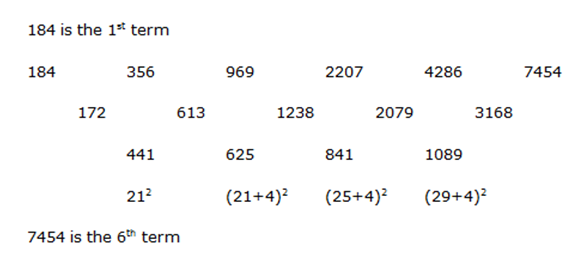Series –II pattern: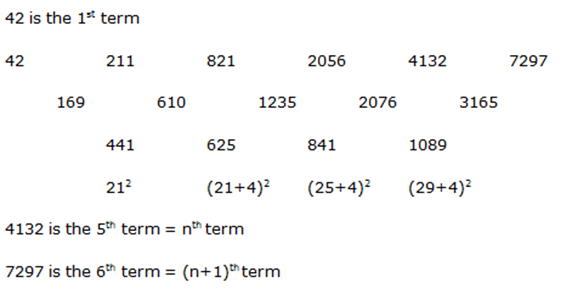Series –I pattern: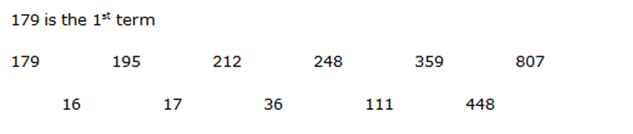Pattern of the difference in the above series is:

16 * 1 + 1 = 17

17 * 2 +2 = 36

36 * 3 + 3 = 111

111 * 4+ 4 = 448

807 is 6th term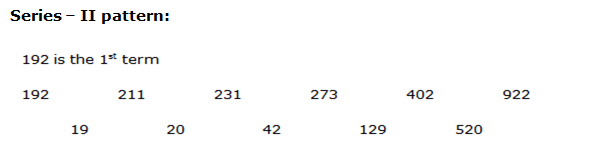Pattern of the difference in the above series is:

19 * 1 + 1 = 20

20 * 2 + 2 = 42

42 * 3+ 3 = 129

129 * 4 + 4 = 520

922 is 6th term

Series –I pattern:

64 is the 1st term

64 * 0.5 + 1 = 33

33 * 1 + 1 = 34

34 * 1.5 + 1 = 52

52 * 2 + 1 = 105

105 * 2.5 + 1 = 263.5 = 6th term

Series –II pattern:

72 is the 1st term

72 * 0.5 + 1 = 37

37 * 1 + 1 = 38

38 * 1.5 + 1 = 58

58 * 2 + 1 = 117 = 5th term = (n-2)th term

117 * 2.5 + 1 = 293.5 = 6th term = (n-1)th term

293.5 * 3 + 1 = 881.5 = 7th term = nth term

Series – I pattern: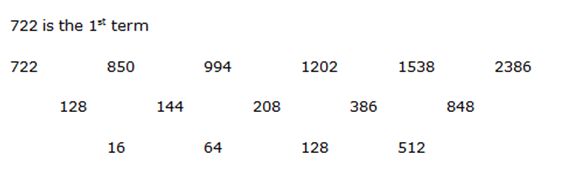Pattern of the difference of difference in the above series is:

16 * 4 = 64

64 * 2 = 128

128 * 4 = 512

2386 is the 6th term

Series –II pattern: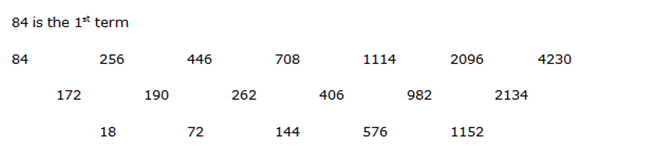Pattern of the difference of difference in the above series is:

18 * 4 = 72

72 * 2 = 144

144 * 4 = 576

576 * 2 = 1152

4230 is the 7th term = nth term

(n +1) = 7 +1 = 8